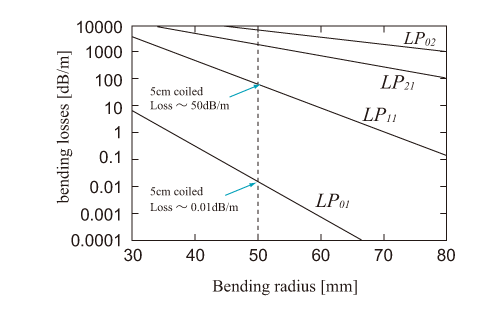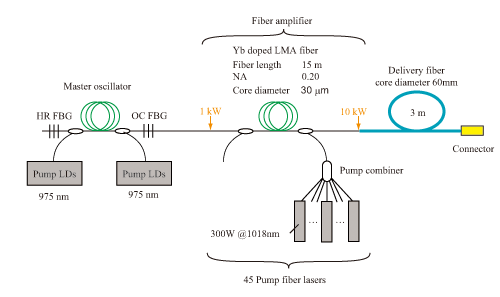In a typical SI-type optical fiber, the spatial mode number, N, is expressed as N~V2/4, provided that the degree of freedom of polarization is excluded. For NA = 0.06, and the wavelength of 1064nm, N is obtained as N=3, 7, 78, and > 300, at the core diameter of d = 20, 30, 100, and 200 µm, respectively.

In the electric field distribution of light with multimode propagation, the higher-order mode leaks from the core to the clad. By bending the optical fiber, the bending loss of higher-order mode is enlarged without increasing the bending loss of fundamental mode. Since NF is as small as 0.06 for an LMA fiber, giving a large curvature can control linear polarization (LP) mode. As a result, a good beam quality (nearly single mode: M2~1) is realized (mode filtering effect).

Coiling an LMA fiber is well-known method for suppressing higher-order modes. Fig. 1 shows the relationship between the curvature radius and bending loss in the initial four transverse modes for the coiled LMA fiber with core diameter of 30 µm and NA = 0.06. For the fiber curvature radius of 5 cm, the bending loss of LP01 mode is ~0.01 dB/m, while the bending loss of LP11 mode is ~50 dB/m. This LMA fiber enables the SM propagation of laser light when it is bend with the curvature radius of 5 cm.Fig 1. Curvature radius and bending loss of optical fiber

The singlemode output power exceeded 1 kW in 2004 (the previous maximal power was 100 W). This achievement attributes to the development of LMA fiber and high power semiconducting laser. Nowadays, 10 kW output is possible by using the setup shown in Fig. 2. 15~20 kW is expected in the future perspective. By using the LMA Yb DCF amplification with the core diameter of 80 µm and NA = 0.06 and suppressing the nonlinear optical effects, SM output with peak power of 1.6 MW, pulse duration of 700 ps, pulse energy of 1 mJ, and repetition rate of 50 Hz was realized.Fig. 2 Configuration of 10 kW singlemode fiber laser system